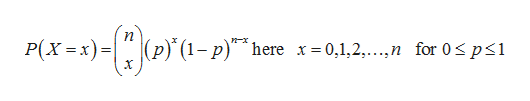# A basketball player has a free throw percentage of 93%. What is the probability she misses at least one of his next 27 free throws?

Question
128 views

A basketball player has a free throw percentage of 93%. What is the probability she misses at least one of his next 27 free throws?

check_circle

Step 1

The probability of she misses at least one of his next 27 free throws is obtained below:

Let X be the number of throws until she the first missed,  93% of player has a free throw, that is q = 0.93. 7% of does not make her free throw, that is p = 0.07 (1–0.93).

Therefore, the distribution is binomial distribution with sample size 27 and probability of success 0.07. That is, X~ Binomial (27, 0.07).

The formula for the probability of binomial distribution is,help_outlineImage TranscriptioncloseAк -те-" "Вove-pт Р(х - х)- р)"" here x %3D0,1,2,....,п for 0sp<1 . ,00)-е fullscreen
Step 2

The required proba...

### Want to see the full answer?

See Solution

#### Want to see this answer and more?

Solutions are written by subject experts who are available 24/7. Questions are typically answered within 1 hour.*

See Solution
*Response times may vary by subject and question.
Tagged in

### Other